Miscellaneous

Chapter 4 Class 11 Complex Numbers
Serial order wise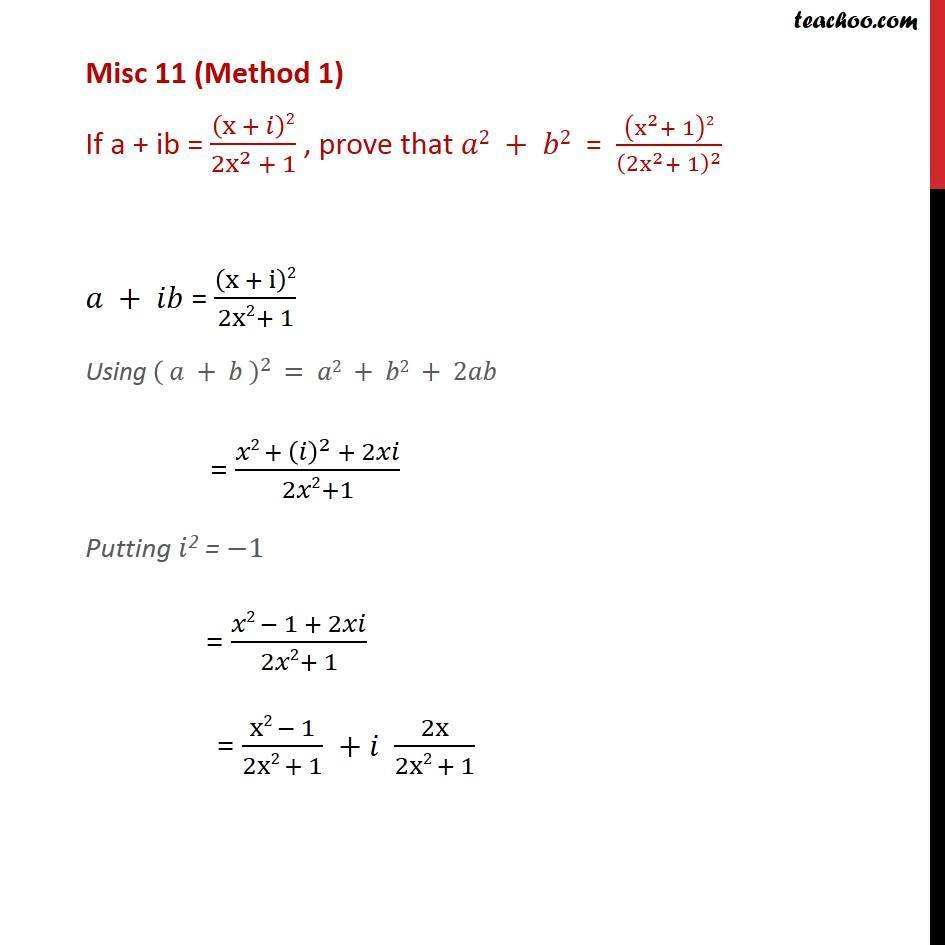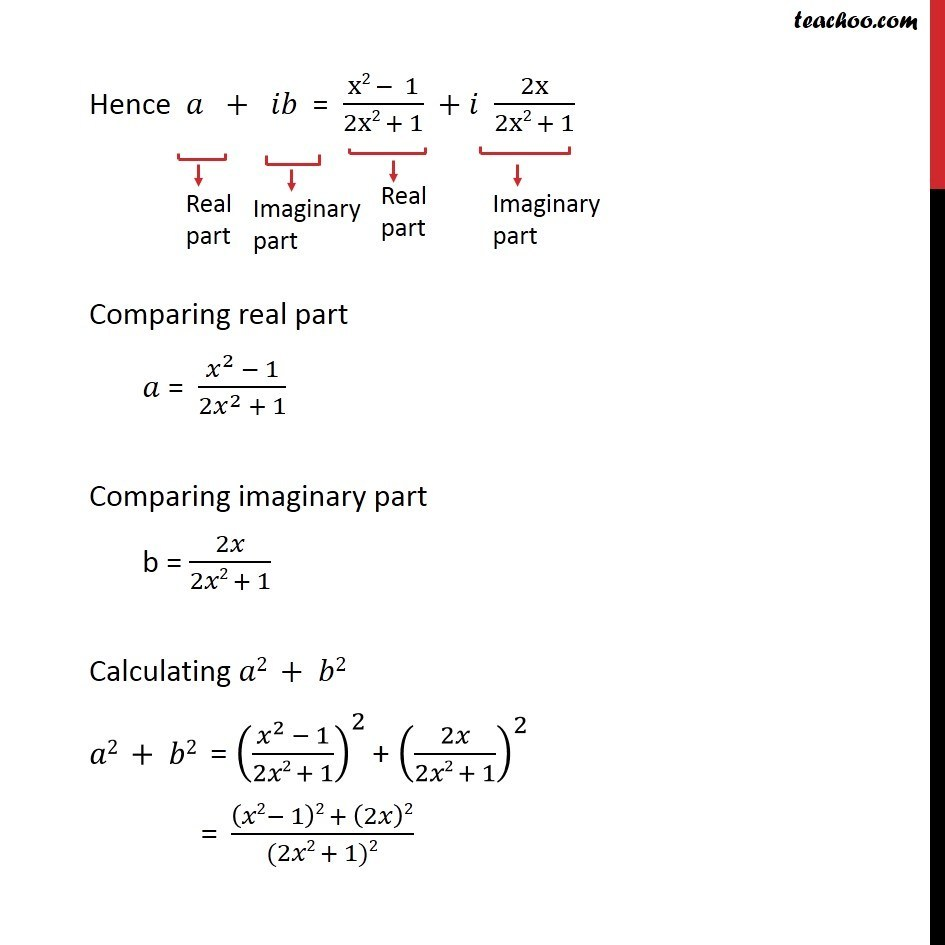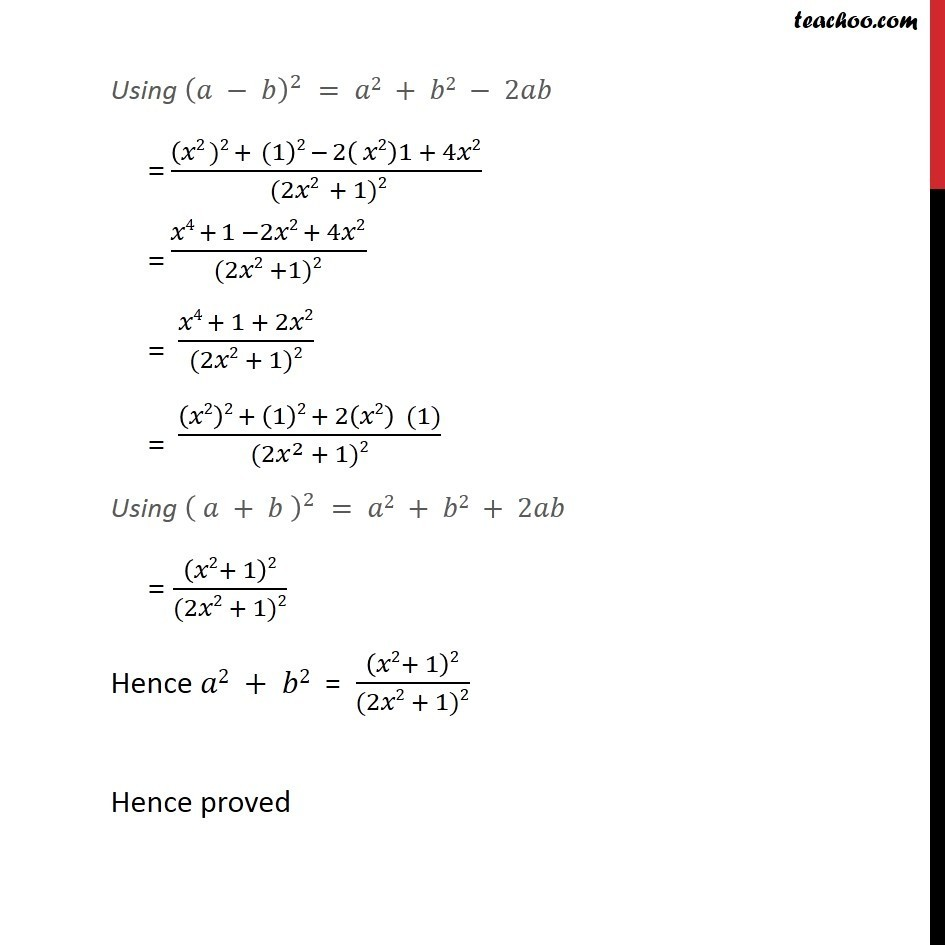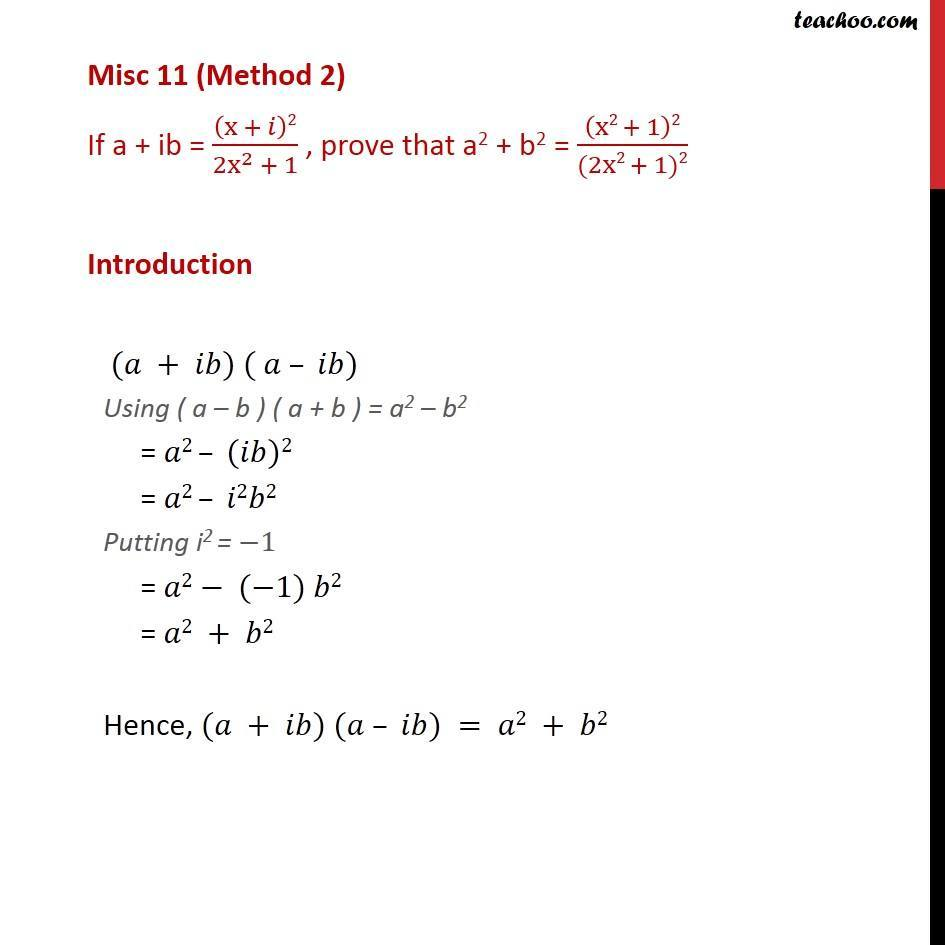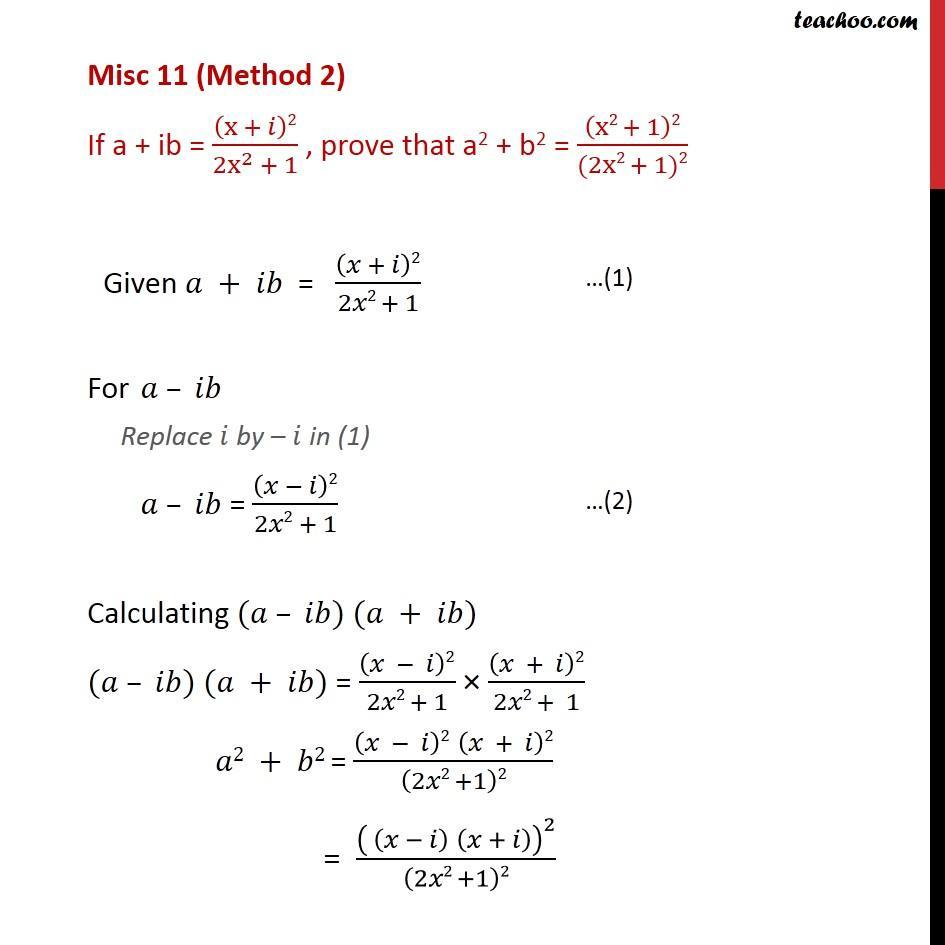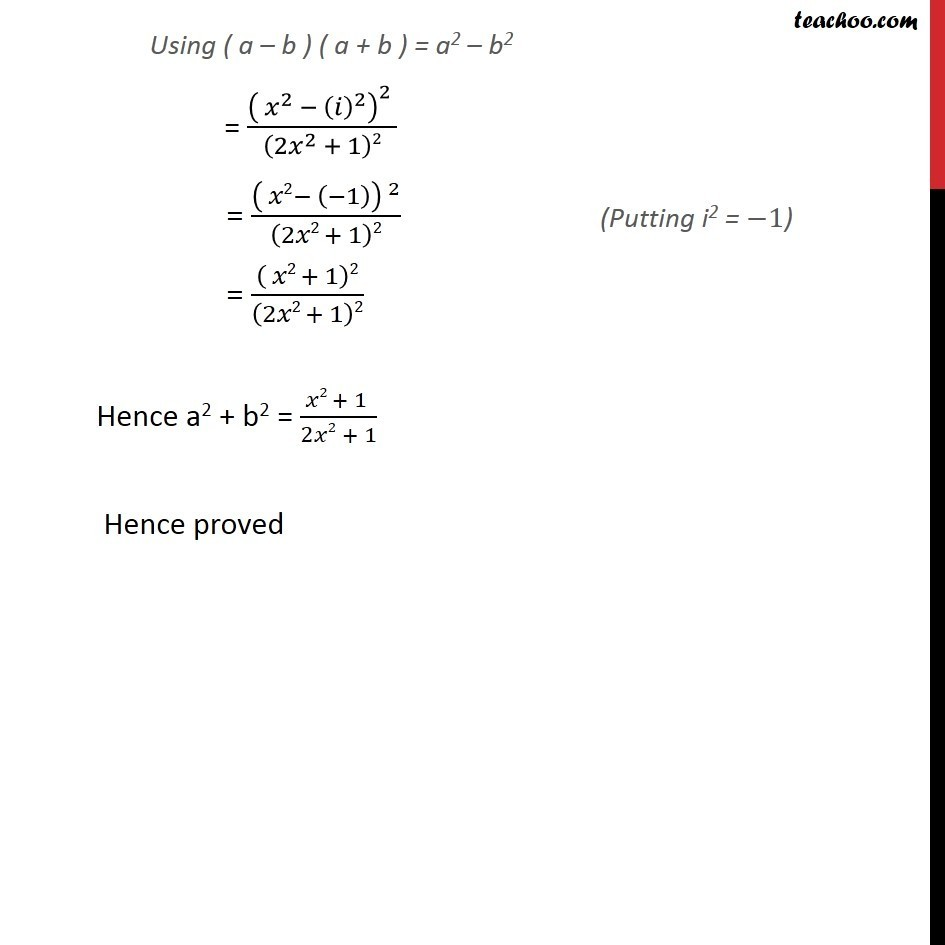Learn in your speed, with individual attention - Teachoo Maths 1-on-1 Class

### Transcript

Misc 6(Method 1) If a + ib = (x + 𝑖)2/(2x^2 + 1) , prove that 𝑎2 + 𝑏2 = (x^2+ 1)2/(2x^2+ 1)^2 𝑎 + 𝑖𝑏 = (x + i)2/(2x2+ 1) Using ( 𝑎 + 𝑏 )^2 = 𝑎2 + 𝑏2 + 2𝑎𝑏 = (𝑥2 + (𝑖)^2 + 2𝑥𝑖)/(2𝑥2+1) Putting 𝑖2 = −1 = (𝑥2 − 1 + 2𝑥𝑖)/(2𝑥2+ 1) = (x2 − 1)/(2x2 + 1) + 𝑖 2x/(2x2 + 1) Hence 𝑎 + 𝑖𝑏 = (x2 − 1)/(2x2 + 1) + 𝑖 2x/(2x2 + 1) Comparing real part 𝑎 = (𝑥^2 − 1)/(2𝑥^2 + 1) Comparing imaginary part b = 2𝑥/(2𝑥2 + 1) Calculating 𝑎2 + 𝑏2 𝑎2 + 𝑏2 = ((𝑥^2 − 1)/(2𝑥2 + 1))^2 + (2𝑥/(2𝑥2 + 1))^2 = ((𝑥2− 1)2 + (2𝑥)2)/((2𝑥2 + 1)2) Using (𝑎 − 𝑏)^2 = 𝑎2 + 𝑏2 − 2𝑎𝑏 = ((𝑥2 )2 + (1)2 − 2( 𝑥2)1 + 4𝑥2)/( (2𝑥2 + 1)2) = (𝑥4 + 1 −2𝑥2 + 4𝑥2)/((2𝑥2 +1)2) = (𝑥4 + 1 + 2𝑥2)/((2𝑥2 + 1)2) = ((𝑥2)2 + (1)2 + 2(𝑥2) (1))/((2𝑥^2 + 1)2) Using ( 𝑎 + 𝑏 )^2 = 𝑎2 + 𝑏2 + 2𝑎𝑏 = (𝑥2+ 1)2/((2𝑥2 + 1)2) Hence 𝑎2 + 𝑏2 = (𝑥2+ 1)2/((2𝑥2 + 1)2) Hence proved Misc 6(Method 2) If a + ib = (x + 𝑖)2/(2x^2 + 1) , prove that a2 + b2 = (x2 + 1)2/((2x2 + 1)2) Introduction (𝑎 + 𝑖𝑏) ( 𝑎 – 𝑖𝑏) Using ( a – b ) ( a + b ) = a2 – b2 = 𝑎2 – (𝑖𝑏)2 = 𝑎2 – 𝑖2𝑏2 Putting i2 = −1 = 𝑎2− (−1) 𝑏2 = 𝑎2 + 𝑏2 Hence, (𝑎 + 𝑖𝑏) (𝑎 – 𝑖𝑏) = 𝑎2 + 𝑏2 Misc 6(Method 2) If a + ib = (x + 𝑖)2/(2x^2 + 1) , prove that a2 + b2 = (x2 + 1)2/((2x2 + 1)2) Given 𝑎 + 𝑖𝑏 = (𝑥 + 𝑖)2/(2𝑥2 + 1) For 𝑎 – 𝑖𝑏 Replace 𝑖 by – 𝑖 in (1) 𝑎 – 𝑖𝑏 = (𝑥 − 𝑖)2/(2𝑥2 + 1) Calculating (𝑎 – 𝑖𝑏) (𝑎 + 𝑖𝑏) (𝑎 – 𝑖𝑏) (𝑎 + 𝑖𝑏) = (𝑥 − 𝑖)2/(2𝑥2 + 1) × (𝑥 + 𝑖)2/(2𝑥2 + 1) 𝑎2 + 𝑏2 = ((𝑥 − 𝑖)2 (𝑥 + 𝑖)2)/(2𝑥2 +1)2 = ( (𝑥 − 𝑖) (𝑥 + 𝑖))^2/(2𝑥2 +1)2 Using ( a – b ) ( a + b ) = a2 – b2 = (( 𝑥^2 − (𝑖)^2 )^2 )/(2𝑥^2 + 1)2 = 〖( 𝑥2− (−1)) 〗^2/(2𝑥2 + 1)2 = ( 𝑥2 + 1)2/(2𝑥2 + 1)2 Hence a2 + b2 = (𝑥2 + 1 )/(2𝑥2 + 1) Hence proved